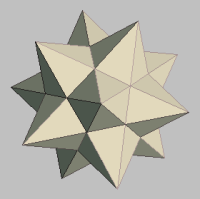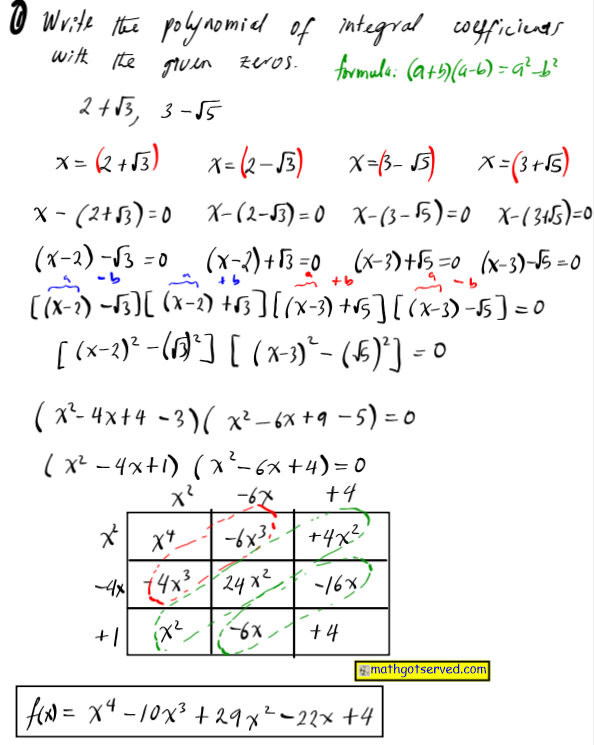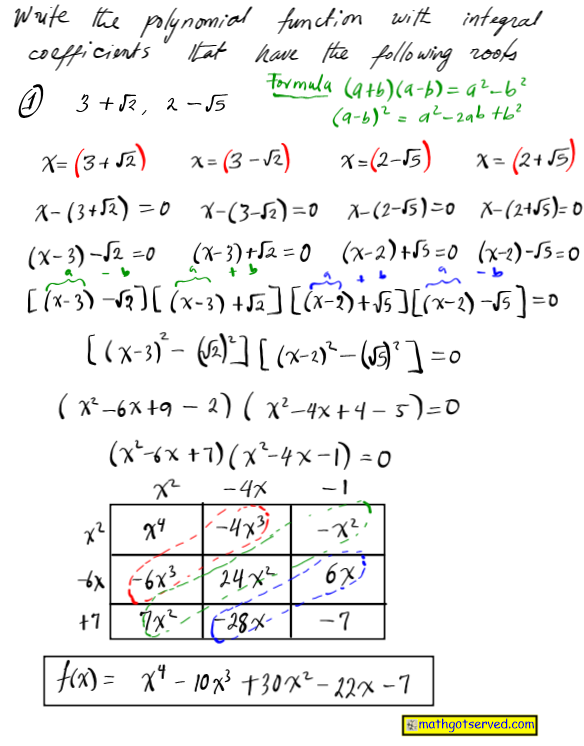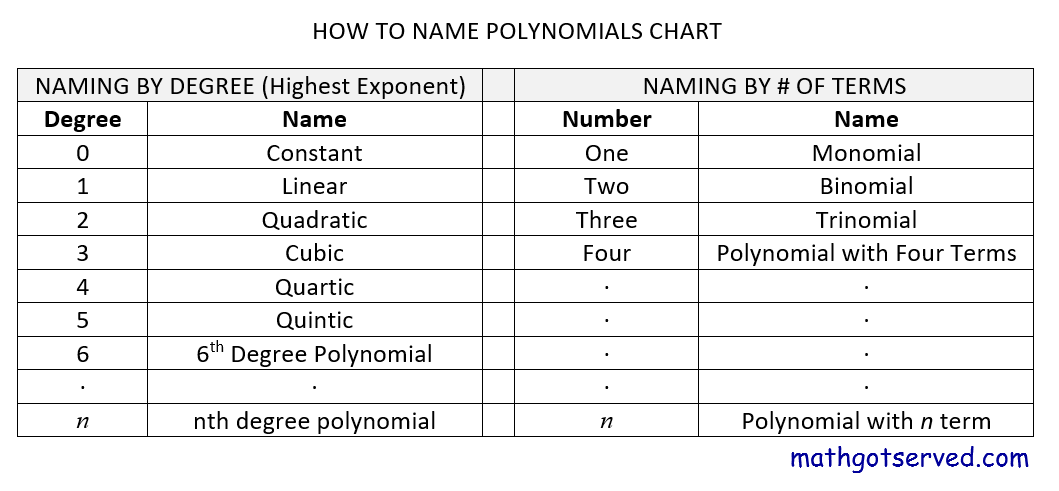MATHGOTSERVEDALGEBRA 2 HONORS
UNIT 3 : POLYNOMIAL FUNCTIONS
Overview of Polynomials
Polynomials:
Definition(meaning what is?): A polynomial function is a function that can be written in the form  f(x)=anxn+an-1xn-1+…a1x+a0
where n is a positive integer and an to a. are real numbers with an not being equal to zero. A polynomial is a sum of difference of monomials which are product of numbers and variables with whole number exponents. Polynomials do not have variable denominators or operations such as absolute values or roots.

Degree of a Polynomial
Definition( meaning of what is) The degree of a polynomial is the value of the exponent of the monomial term with the highers degree which is the sum of the exponents of the variables of that monomial.

Naming Polynomials by Degree
Degree 1 (Linear Function ): A polynomial of degree 1 is known as a linear function. It can be written in the form y=mx+b
Degree 2 (Quadratic Function): A polynomial of degree 2 is  known as a quadratic function. The quadratic function can be written in different forms. Below are the forms of a quadratic function
Standard form y=ax^2+bx+c  (Best form for finding the y-intercept=c)
Vertex form y=a[b(x-h)]^2+k  (Best form for finding the vertex and consequently the shifts.
Degree 3(Cubic Functions) A polynomial of degree 3 is know as a cubic function

2-2 FACTORING POLYNOMIALS
VIDEO TUTORIAL ON FACTORING POLYNOMIALS
2-2 POLYNOMIAL FUNCTIONS OF HIGHER DEGREES

Polynomials of higher degrees can be generated when you are provided with sufficient information concerning the zeros.

VIDEO TUTORIAL ON WRITING POLYNOMIALS WITH GIVEN ROOTS
2-3 RATIONAL ROOTS LONG &SYNTHETIC  DIVISION REMAINDER THEOREM
VIDEO TUTORIAL ON OPERATION ON FUNCTIONS
GALLERY OF SOLVED PROBLEMS
1.2.3.2-4 COMPLEX NUMBERS OPERATIONS
2-5 COMPLEX NUMBERS OPERATIONS
2-5 COMPLEX NUMBERS OPERATIONS